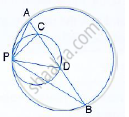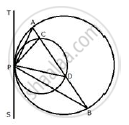Share

# Two Circles Touch Each Other Internally at a Point P. a Chord Ab of the Bigger Circle Intersects the Other Circle in C and D. Prove that ∠Cpa = ∠Dpb. - Mathematics

Course

#### Question

Two circles touch each other internally at a point P. A chord AB of the bigger circle intersects the other circle in C and D. Prove that ∠CPA = ∠DPB.#### SolutionDraw a tangent TS at P to the circles given.
Since TPS is the tangent, PD is the chord.
∴ ∠PAB = ∠BPS ……..(i) (Angles in alternate segment)
Similarly,
∠PCD = ∠DPS …… (ii)
Subtracting (i) from (ii)
∠PCD - ∠PAB = ∠DPS - ∠BPS
But in ∠PAC,
Ext. ∠PCD = ∠PAB + ∠CPA
∴ ∠PAB + ∠CPA - ∠PAB  = ∠DPS - ∠BPS
⇒ ∠CPA  = ∠DPB

Is there an error in this question or solution?
Two Circles Touch Each Other Internally at a Point P. a Chord Ab of the Bigger Circle Intersects the Other Circle in C and D. Prove that ∠Cpa = ∠Dpb. Concept: Tangent Properties - If Two Circles Touch, the Point of Contact Lies on the Straight Line Joining Their Centers.